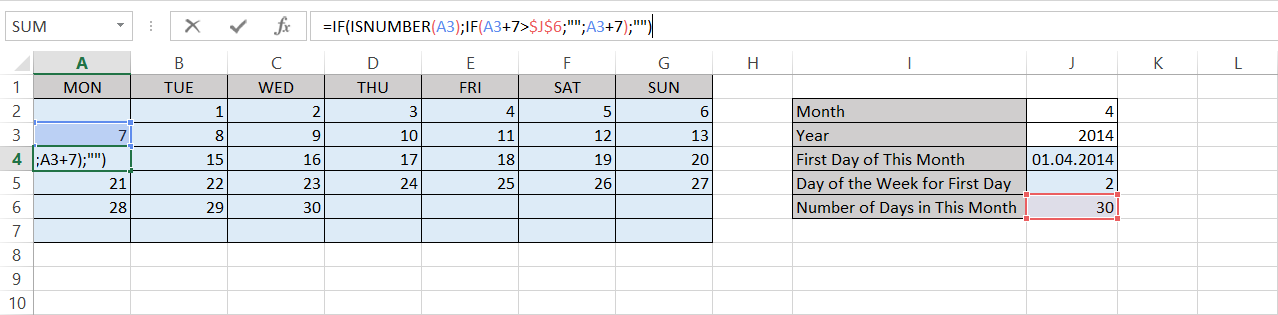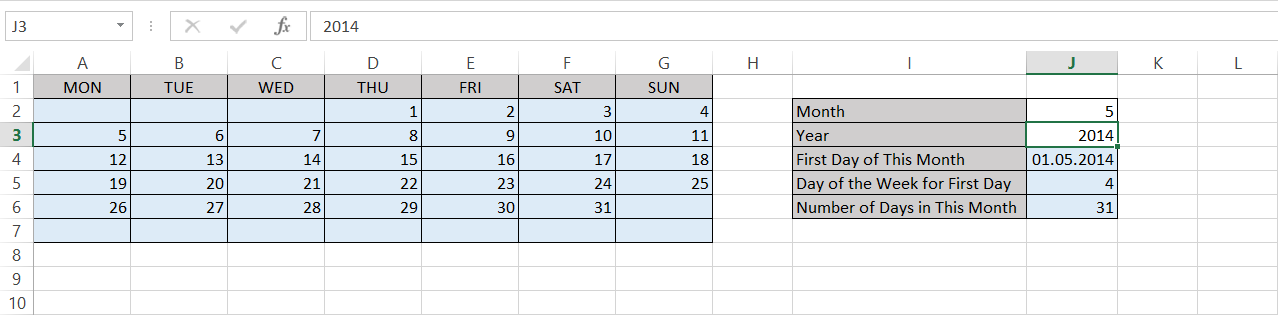## Thursday, May 1, 2014

### Calendar Template in Excel

I like keeping track of things I do. Hence, I have a list of books I read, I keep a journal at work and for social life I have a calendar where I take notes for events, dinners, parties, meetings … etc. Before I started using my current calendar, I tried finding a customizable calendar. But I couldn’t find any. Then I thought it might be better to create my own using Excel.

At the end of this post, you can create your own Excel calendar template. Let's start with the inputs...Figure 1

Above you can see that there is a data table at the right hand side of the calendar. The fields are described below:

• Month: Input field for the month you want to have a calendar for (1 to 12)
• Year: Input field for the year you want to have a calendar for (1901 to 9999)
• First Day of This Month: Calculated field with this formula: =DATE(J3;J2;1)
• Day of the Week for First Day: Calculated field with this formula: =WEEKDAY(J4;2)
• Number of Days in This Month: Calculated field with this formula: =DAY(DATE(J3;J2+1;1)-1)

As you can see, the names of days in a week are listed through cell A1 to G1 manually. If you want to week to start with Sunday, then you need to change WEEKDAY formula above (For details, you can refer to Excel WEEKDAY function)

The day numbers start from the second row. In the function bar, you can see how the days are printed with this function: =IF(\$J\$5=COLUMN();1;IF(INDEX(\$A\$2:\$G\$2;1;\$J\$5)+(COLUMN()-\$J\$5)>1;INDEX(\$A\$2:\$G\$2;1;\$J\$5)+(COLUMN()-\$J\$5);"")) You can click and drag formula to the other cells on the same row.Figure 2

Figure 2 shows the second week of the month. Here the days are calculated using the first week’s information with this formula: =IF(ISNUMBER(A2);A2+7;\$G\$2+COLUMN()) You can again click and drag the formula to the other cells on the same row.Figure 3

In Figure 3, the cells are checking the end of month and if it is not the end of month, they are calculating the days using the previous week’s information with this formula: =IF(ISNUMBER(A3);IF(A3+7>\$J\$6;"";A3+7);"") Now, you can click and drag the same formula to the other cells on the same row and other cells on the same column. This will be our last formula.Figure 4

The last figure shows how the calendar changes when we change the input values for month and year.

I hope this helps you. You can customize the cells, change the colour, font and size to get what you need.